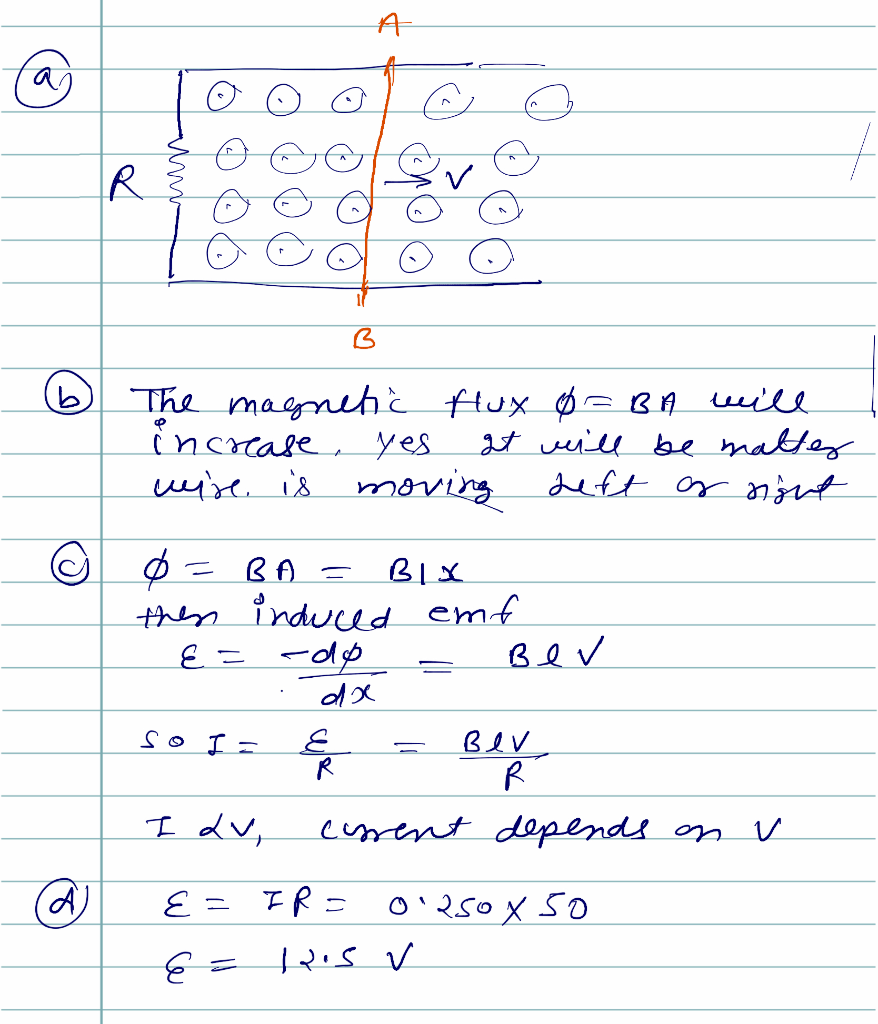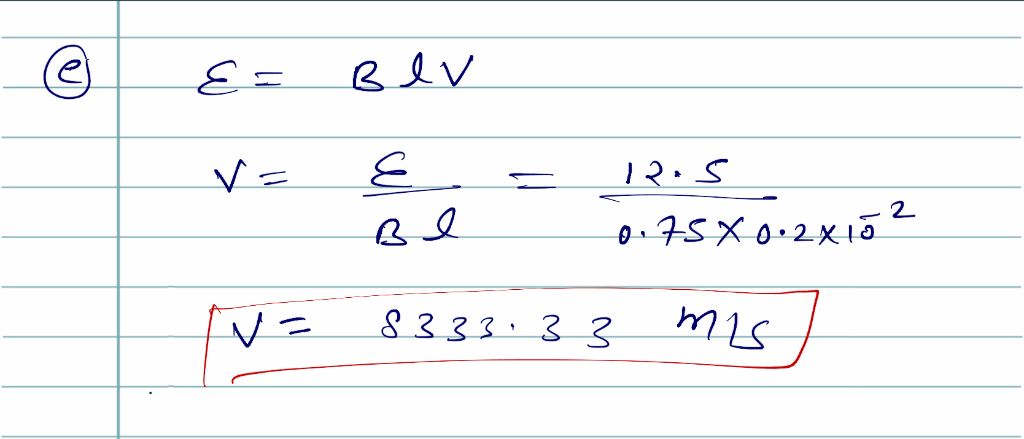Homework Help Question & Answers

A rectangular loop of wire has a resistor with a resistance R=50.00 Ω on the left vertical wire, ...

A rectangular loop of wire has a resistor with a resistance R=50.00 Ω on the left vertical wire, while the light vertical wire can be. over back and forth to make the loop larger or smaller. The vertical wires (the light of the rectangle) are 0.200 cm in length, and the entire circuit is in an area of space where a magnetic field of magnitude 0.750T and pointing out of the page is present.

a) Draw the situation, including the magnetic field, and indicate which wire can move.

b) As the movable wire moves, what will happen to the magnetic field flux through the entire circuit? Does it matter in what direction the wire moves? Explain

c) If a current is to be included in the circuit, does it matter hoes fast the wire moves? explain why or why not?

d) If a current pf 0.250 A is desired across the resistor, what voltage must be present across the resistor?

e) Find the speed at which the movable wire must move in order to include the voltage found in the previous question?

The magnetic flux induced emf and velocityAdd Answer of: A rectangular loop of wire has a resistor with a resistance R=50.00 Ω on the left vertical wire, ...
More Homework Help Questions Additional questions in this topic.

• Part B (Advanced) The figure below shows a long straight wire (Wire 1) carrying a constant current, I. A second wire (Wire 2), carrying a constant current, 12, is placed near the first wire with dime...

Need Online Homework Help?

Get FREE EXPERT Answers
WITHIN MINUTES
Related Questions